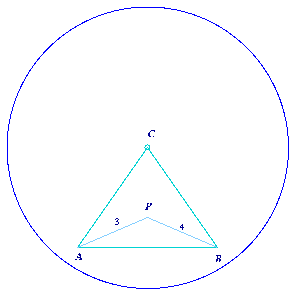#### You may also like### Kissing Triangles

Determine the total shaded area of the 'kissing triangles'.### Isosceles

Prove that a triangle with sides of length 5, 5 and 6 has the same area as a triangle with sides of length 5, 5 and 8. Find other pairs of non-congruent isosceles triangles which have equal areas.### Trice

ABCDEFGH is a 3 by 3 by 3 cube. Point P is 1/3 along AB (that is AP : PB = 1 : 2), point Q is 1/3 along GH and point R is 1/3 along ED. What is the area of the triangle PQR?

# Triangle Inequality

##### Age 11 to 14 Challenge Level:

Several students from West Flegg worked on this one by drawing . We apologise to those who were annoyed because the number 10 did not seem to come into the solution.

The triangle inequality states: "the length of one side of a triangle is always less than the sum of the lengths of the other two sides of the triangle". Draw a diagram and convince yourself that this has to be true.

So, using the triangle inequality, AB < AP + PB = 3 + 4 = 7 .

As triangle ABC is equilateral we also know now that CA < 7 and CB < 7. Now applying the triangle inequality to triangle CAP, and using the fact that CA < 7, gives CP < CA + AP < 7 + 3 = 10.

This shows that CP is less than 10 cm but it is obviously quite a lot less than 10 cm.

To get a better estimate, draw a circle, centre C, radius 7 centimetres, then since CA and CB are both less than 7 centimetres the whole of triangle ABC is inside the circle. As point P is inside triangle ABC it must be inside the circle and hence CP < 7 cm.